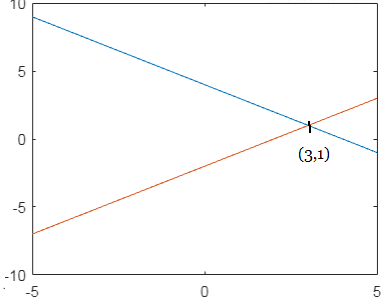# Solve the system of equations by graphing. x + y = 4 x - y = 2

## Question:

Solve the system of equations by graphing.

{eq}x + y = 4 \\ x - y = 2 {/eq}

## Graphical Method:

To solve the system of two given equations using the graphical method, we just graph both the equations and see where they meet. The point of intersection is the solution to the system.

The given equations are:

\begin{align} x+y=4 & \Rightarrow y= -x+4 \\ x-y=2& \Rightarrow y= x-2 \end{align}

We graph both equations.The point of intersection of both lines is {eq}(3,1) {/eq}.

So the solution of the given system is: {eq}\boxed{\mathbf{x=3; \, y=1}} {/eq}.